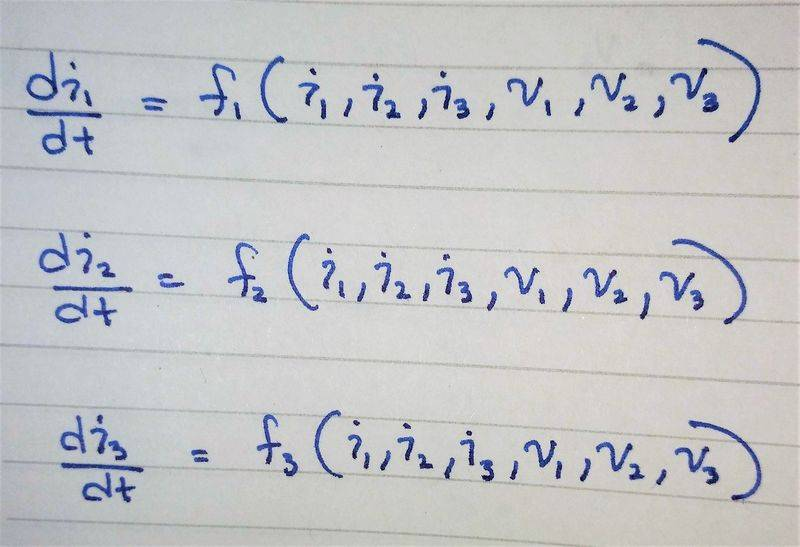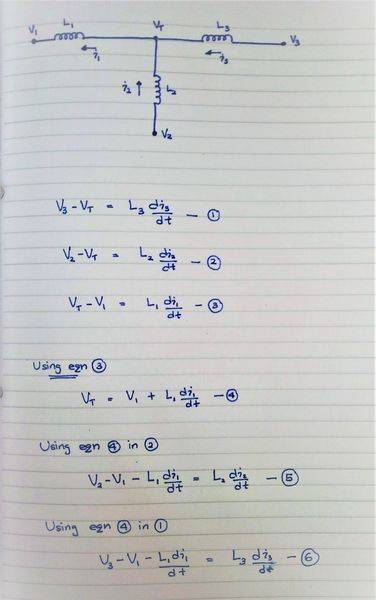# Equations for three inductors connected together

• anon6912
In summary, you eliminated the intermediary variable VT and wrote the state equations in terms of V1 V2 V3 and I1 and I2.f

#### anon6912

I have a system with three inductors connected together at a common point.
The unconnected ends of each inductor is connected to an independent voltage source.

Basically I want to get three expressions for the dynamics of the currents with V1, V2 and V3 as inputs.
i.e. i need to eliminate the intermediary variable VT.

I want to write the state equations for the system in the following format (state space form):The system and, how far i got is below:I don't know how to proceed from here.
Or is it impossible to get it into the format I want?

#### Attachments

i.e. i need to eliminate the intermediary variable VT.

The system and, how far i got is below:Here you removed ##V_{T}## from all the equations. You have di/dt (for 1, 2 and 3) in terms of V1,V2... etc without ##VT##.

Maybe I didn't understand your question correctly.

#### Attachments

You are missing an equation. Using the sign conventions from your drawing, i1=i2+i3. That is Kirchoff's Current Law.

•Fisherman199
Here you removed ##V_{T}## from all the equations. You have di/dt (for 1, 2 and 3) in terms of V1,V2... etc without ##VT##.

Maybe I didn't understand your question correctly.

At the time I was asking this question I don't think I had grasped the question correctly even myself.

What I initially wanted was to eliminate V_T.
But as a consequence of doing this I found out I had to/ could write I_1 in terms of I2 and I3.
I wasn't sure if this second part of replacing I1 with !2 and I3 was correct because I felt like it would eliminate a state from the system if I did that.
But I think it is correct and can be done.

So now I have a system with VT eliminated and the equations written in terms of V1 V2 V3 and I1 and I2 :)

•Phylosopher
You are missing an equation. Using the sign conventions from your drawing, i1=i2+i3. That is Kirchoff's Current Law.
Thanks. I ended up integrating kirchhoff law and it worked :)